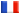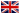# Séminaire de Cryptographie

## Clauss Diem### On the Subexponentiality of the Elliptic Curve Discrete Logarithm Problem over Extension Fields

The purpose of the talk is to present the following heuristic result.

Let a, b in R with 0 < a < b. Then discrete logarithms in E(F_q^n), where q is a prime power, a log_2(q) \leq n \leq b \log_2(q)\$ and E/F_q^n is any elliptic curve over F_q^n, can be solved in probabilistic subexponential time L[3/4].

The algorithm is a variant of a recent index calculus algorithm by Gaudry. The main difference is that we increase the factor base.